# 对超弹性材料应用损伤演化定律

Guest
2020年 9月 8日

### 损伤模型的数学背景

\dot{\omega} =-\beta \omega e ^{(\frac{b \omega \psi}{RT})},

k=Ae^{(\frac{-E_b}{RT})}

k=Ae^{(-\frac{E_b-b\omega\psi}{RT})} = Ae^{(-\frac{E_b}{RT})}e^{(\frac{b\omega\psi}{RT})} = \beta e ^{(\frac{b\omega\psi}{RT})}.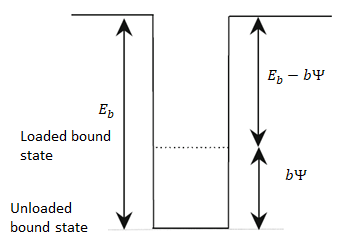D_{int}=\frac{1}{2}\mathbf{S}:\mathbf{\dot{C}}-\mathbf{\dot{\psi}}=\frac{1}{2}\mathbf{S}:\mathbf{\dot{C}}-(\frac{\partial\psi}{\partial\mathbf{C}}:\mathbf{\dot{C}}+\frac{\partial\psi}{\partial\omega}\dot{\omega})=-\frac{\partial\psi}{\partial\omega}\dot{\omega}\geq0.

### 在 COMSOL Multiphysics® 中模拟损伤模型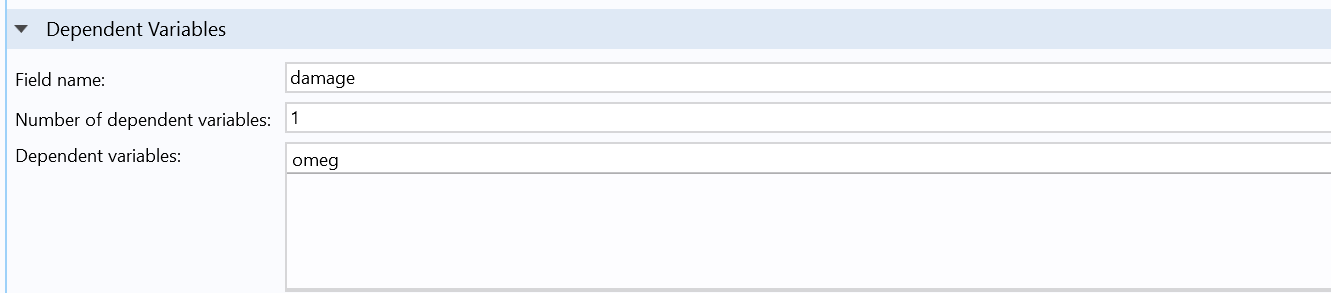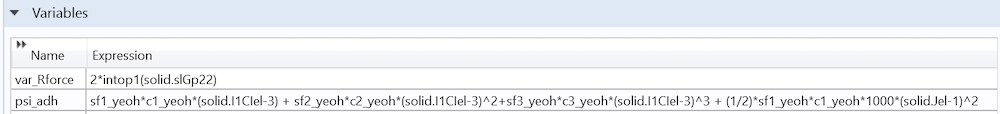### 结果：优化有损伤的超弹性模型的材料参数

#### 优化参数和力-位移曲线

s_{f1yeoh} 9.980 10.62 \beta 3.27e-2 [1/s] 4.24e-2 [1/s]
s_{f2yeoh} 0.366 0.403 b 4.81e-4 [m 3 /mol] 4.12e-4 [m 3 /mol] = 0.41/M
s_{f3yeoh} 0.260 0.203

121570#### 应变率依赖性#### 滞后和材料循环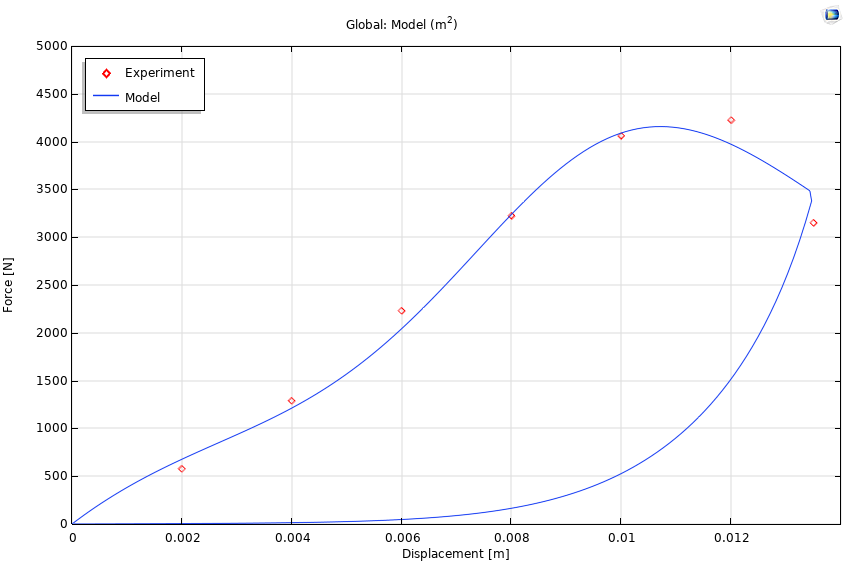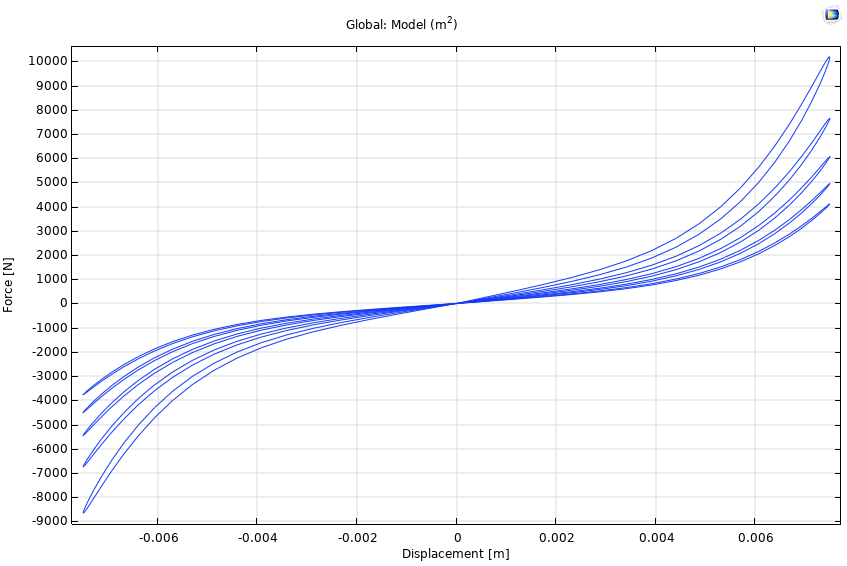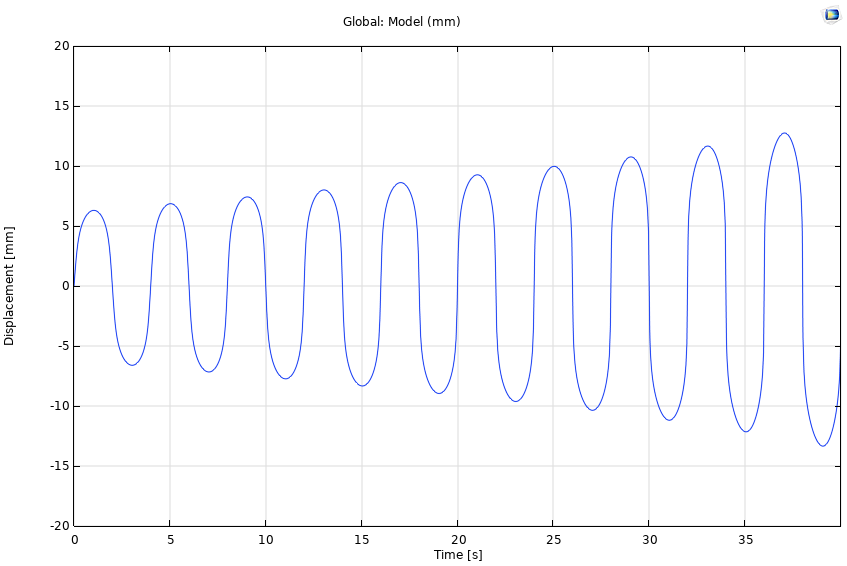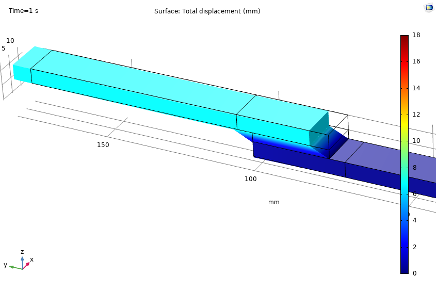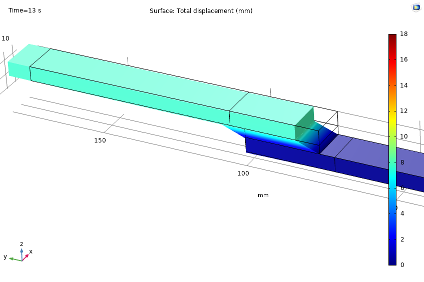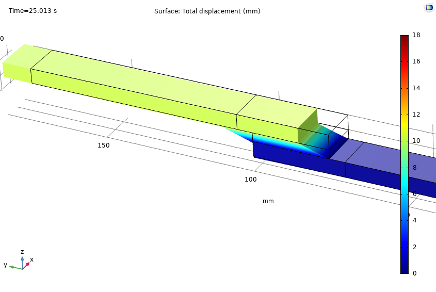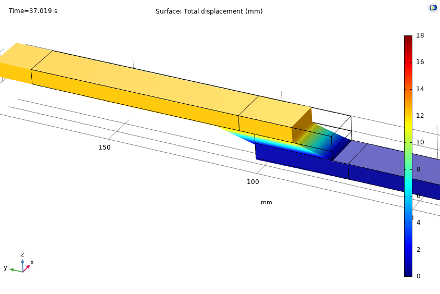\dot{\omega}=
\begin{cases}
\end{cases}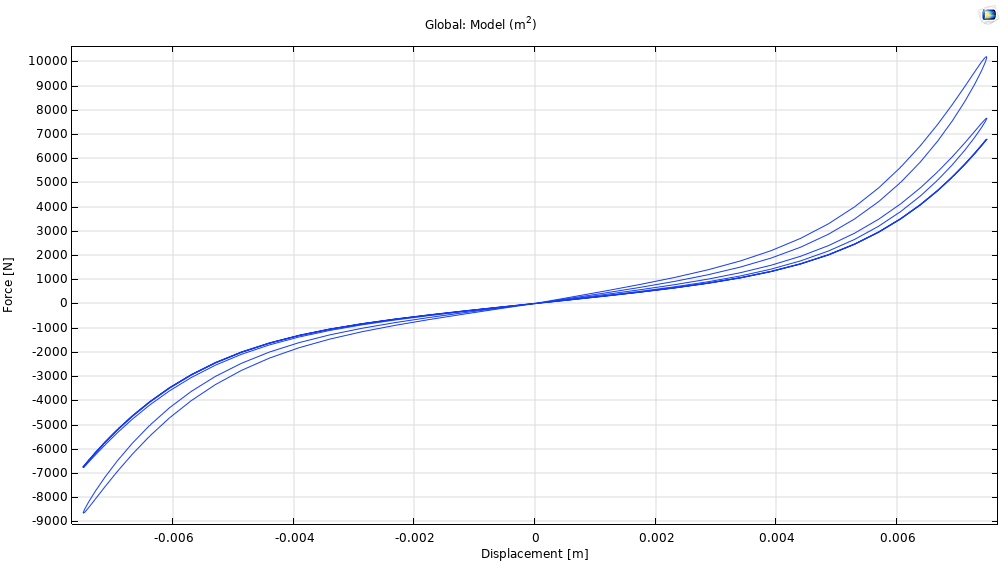• 滞后性
• 单调加载期间的材料软化/破裂
• 循环材料软化
• 应变率相关性
• 蠕变性

### 关于作者

Björn Fallqvist 是 Lightness by Design 公司的一名顾问，从事基于数值分析的产品开发工作。他于 2016 年获皇家理工学院博士学位，致力于开发用于捕捉生物细胞机械行为的本构模型。主要研究兴趣是材料表征领域，专长是和使用各种材料模型捕捉物理现象。

1. B. Fallqvist, M. Kroon, “Constitutive modelling of composite biopolymer networks”, Stockholm: Journal of Theoretical Biology, vol. 395, 2016.
2. B. Fallqvist, M. Kroon, “A chemo-mechanical constitutive model for cross-linked actin networks and a theoretical assessment of their viscoelastic behaviour”, 2, Stockholm: Biomechanics and Modeling in Mechanobiology, vol. 12, 2013.